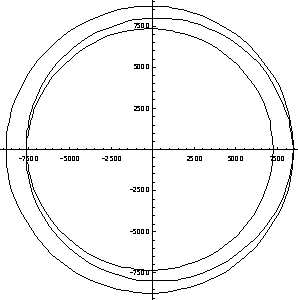# Sketch of a Proof by Birkhoff and MacLane

Consider the polynomial P(z) as a mapping from one copy of the complex plane (say, the z-plane) to another copy of the complex plane (say, the w-plane). Such a mapping transforms a circle |z| = r of radius r into a closed curve on the w-plane (see Figure 1). For very large values of r, the anz n term dominates, and the image is a closed curve which loops n times around the origin of the w-plane (see Figure 2). On the other hand, for very small values of r, we may neglect all the terms except amz m + a0, where am is the coefficient with the lowest index m>0 which is not equal to zero, and the image loops m times around the point w = a0 (Figure 3). As r0, the image collapses to the point a0. We now invoke the topological fact that if we start with r very large, and continuously reduce r to 0, simultaneously reducing the image in the w -plane from a large loop encircling the origin (likely more than once) to a point, we must necessarily encounter a stage where the image curve passes through the origin w = 0. Thus, we have shown that some point on the z-plane maps to w = 0 - that is, that there is a complex root of P(z). (For details, see Birkhoff & MacLaine, A Survey of Modern Algebra, AK Peters, 1997)Figure 1. Image of circle |z| = 2 under the mapping P(z) = z3 - 2z2 + z - 1. Figure 2. Image of circle |z| = 20 under the mapping P(z) = z3 - 2z2 + z - 1.Figure 3. |z| = 0.1 under the mapping P(z) = z3 - 2z2 + z - 1. Note the enlarged scale. Figure 4. Image of circle |z| = 0.75 under the mapping P(z) = z3 - 2z2 + z - 1. Apparently a root of this polynomial lies very near this circle.